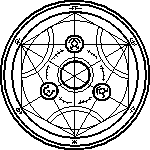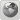CrimsonDiabolics Studios
Would you like to react to this message? Create an account in a few clicks or log in to continue.

# COD 9: Project IRON WOLF? Black Ops 2?

Page 5 of 5##Re: COD 9: Project IRON WOLF? Black Ops 2?

_________________Nazi Zombie Rounds
Nacht Der Untoten: [✓] 10+ [✓] 20+ [] 30+ [] 40+ Multi/Solo
Zombie Verruct: [✓] 10+ [✓] 20+ [] 30+ [] 40+ Multi/Solo
Shi No Numa: [✓] 10+ [✓] 20+ [] 30+ [] 40+ Multi/Solo
Der Riese: [✓] 10+ [✓] 20+ [✓] 30+ [] 40+ Multi/Solo
Kino Der Toten: [✓] 10+ [✓] 20+ [✓] 30+ [✓] 40+  [] 50+ Multi/Solo
FIVE: [✓] 10+ [✓] 20+ [] 30+ [] 40+ Multi/Solo
Ascension: [✓] 10+ [✓] 20+ [✓] 30+ [] 40+ Multi/Solo
Call of the Dead: [✓] 10+ [✓] 20+ [] 30+ [] 40+ Multi/Solo
Shangri La:  [✓] 10+ [✓] 20+ [✓] 30+ [] 40+ Multi/Solo
MOON: [✓] 10+ [✓] 20+ [] 30+ [] 40+ Multi/SoloCDReborn
FounderPosts : 379
Reputation : 3
Join date : 2011-02-02
Age : 26##Re: COD 9: Project IRON WOLF? Black Ops 2?

_________________Nazi Zombie Rounds
Nacht Der Untoten: [✓] 10+ [✓] 20+ [] 30+ [] 40+ Multi/Solo
Zombie Verruct: [✓] 10+ [✓] 20+ [] 30+ [] 40+ Multi/Solo
Shi No Numa: [✓] 10+ [✓] 20+ [] 30+ [] 40+ Multi/Solo
Der Riese: [✓] 10+ [✓] 20+ [✓] 30+ [] 40+ Multi/Solo
Kino Der Toten: [✓] 10+ [✓] 20+ [✓] 30+ [✓] 40+  [] 50+ Multi/Solo
FIVE: [✓] 10+ [✓] 20+ [] 30+ [] 40+ Multi/Solo
Ascension: [✓] 10+ [✓] 20+ [✓] 30+ [] 40+ Multi/Solo
Call of the Dead: [✓] 10+ [✓] 20+ [] 30+ [] 40+ Multi/Solo
Shangri La:  [✓] 10+ [✓] 20+ [✓] 30+ [] 40+ Multi/Solo
MOON: [✓] 10+ [✓] 20+ [] 30+ [] 40+ Multi/SoloCDReborn
FounderPosts : 379
Reputation : 3
Join date : 2011-02-02
Age : 26##Re: COD 9: Project IRON WOLF? Black Ops 2?

_________________Nazi Zombie Rounds
Nacht Der Untoten: [✓] 10+ [✓] 20+ [] 30+ [] 40+ Multi/Solo
Zombie Verruct: [✓] 10+ [✓] 20+ [] 30+ [] 40+ Multi/Solo
Shi No Numa: [✓] 10+ [✓] 20+ [] 30+ [] 40+ Multi/Solo
Der Riese: [✓] 10+ [✓] 20+ [✓] 30+ [] 40+ Multi/Solo
Kino Der Toten: [✓] 10+ [✓] 20+ [✓] 30+ [✓] 40+  [] 50+ Multi/Solo
FIVE: [✓] 10+ [✓] 20+ [] 30+ [] 40+ Multi/Solo
Ascension: [✓] 10+ [✓] 20+ [✓] 30+ [] 40+ Multi/Solo
Call of the Dead: [✓] 10+ [✓] 20+ [] 30+ [] 40+ Multi/Solo
Shangri La:  [✓] 10+ [✓] 20+ [✓] 30+ [] 40+ Multi/Solo
MOON: [✓] 10+ [✓] 20+ [] 30+ [] 40+ Multi/SoloCDReborn
FounderPosts : 379
Reputation : 3
Join date : 2011-02-02
Age : 26##Re: COD 9: Project IRON WOLF? Black Ops 2?

_________________Nazi Zombie Rounds
Nacht Der Untoten: [✓] 10+ [✓] 20+ [] 30+ [] 40+ Multi/Solo
Zombie Verruct: [✓] 10+ [✓] 20+ [] 30+ [] 40+ Multi/Solo
Shi No Numa: [✓] 10+ [✓] 20+ [] 30+ [] 40+ Multi/Solo
Der Riese: [✓] 10+ [✓] 20+ [✓] 30+ [] 40+ Multi/Solo
Kino Der Toten: [✓] 10+ [✓] 20+ [✓] 30+ [✓] 40+  [] 50+ Multi/Solo
FIVE: [✓] 10+ [✓] 20+ [] 30+ [] 40+ Multi/Solo
Ascension: [✓] 10+ [✓] 20+ [✓] 30+ [] 40+ Multi/Solo
Call of the Dead: [✓] 10+ [✓] 20+ [] 30+ [] 40+ Multi/Solo
Shangri La:  [✓] 10+ [✓] 20+ [✓] 30+ [] 40+ Multi/Solo
MOON: [✓] 10+ [✓] 20+ [] 30+ [] 40+ Multi/SoloCDReborn
FounderPosts : 379
Reputation : 3
Join date : 2011-02-02
Age : 26##Re: COD 9: Project IRON WOLF? Black Ops 2?

_________________Nazi Zombie Rounds
Nacht Der Untoten: [✓] 10+ [✓] 20+ [] 30+ [] 40+ Multi/Solo
Zombie Verruct: [✓] 10+ [✓] 20+ [] 30+ [] 40+ Multi/Solo
Shi No Numa: [✓] 10+ [✓] 20+ [] 30+ [] 40+ Multi/Solo
Der Riese: [✓] 10+ [✓] 20+ [✓] 30+ [] 40+ Multi/Solo
Kino Der Toten: [✓] 10+ [✓] 20+ [✓] 30+ [✓] 40+  [] 50+ Multi/Solo
FIVE: [✓] 10+ [✓] 20+ [] 30+ [] 40+ Multi/Solo
Ascension: [✓] 10+ [✓] 20+ [✓] 30+ [] 40+ Multi/Solo
Call of the Dead: [✓] 10+ [✓] 20+ [] 30+ [] 40+ Multi/Solo
Shangri La:  [✓] 10+ [✓] 20+ [✓] 30+ [] 40+ Multi/Solo
MOON: [✓] 10+ [✓] 20+ [] 30+ [] 40+ Multi/SoloCDReborn
FounderPosts : 379
Reputation : 3
Join date : 2011-02-02
Age : 26##Re: COD 9: Project IRON WOLF? Black Ops 2?

_________________Nazi Zombie Rounds
Nacht Der Untoten: [✓] 10+ [✓] 20+ [] 30+ [] 40+ Multi/Solo
Zombie Verruct: [✓] 10+ [✓] 20+ [] 30+ [] 40+ Multi/Solo
Shi No Numa: [✓] 10+ [✓] 20+ [] 30+ [] 40+ Multi/Solo
Der Riese: [✓] 10+ [✓] 20+ [✓] 30+ [] 40+ Multi/Solo
Kino Der Toten: [✓] 10+ [✓] 20+ [✓] 30+ [✓] 40+  [] 50+ Multi/Solo
FIVE: [✓] 10+ [✓] 20+ [] 30+ [] 40+ Multi/Solo
Ascension: [✓] 10+ [✓] 20+ [✓] 30+ [] 40+ Multi/Solo
Call of the Dead: [✓] 10+ [✓] 20+ [] 30+ [] 40+ Multi/Solo
Shangri La:  [✓] 10+ [✓] 20+ [✓] 30+ [] 40+ Multi/Solo
MOON: [✓] 10+ [✓] 20+ [] 30+ [] 40+ Multi/SoloCDReborn
FounderPosts : 379
Reputation : 3
Join date : 2011-02-02
Age : 26##Re: COD 9: Project IRON WOLF? Black Ops 2?

_________________Nazi Zombie Rounds
Nacht Der Untoten: [✓] 10+ [✓] 20+ [] 30+ [] 40+ Multi/Solo
Zombie Verruct: [✓] 10+ [✓] 20+ [] 30+ [] 40+ Multi/Solo
Shi No Numa: [✓] 10+ [✓] 20+ [] 30+ [] 40+ Multi/Solo
Der Riese: [✓] 10+ [✓] 20+ [✓] 30+ [] 40+ Multi/Solo
Kino Der Toten: [✓] 10+ [✓] 20+ [✓] 30+ [✓] 40+  [] 50+ Multi/Solo
FIVE: [✓] 10+ [✓] 20+ [] 30+ [] 40+ Multi/Solo
Ascension: [✓] 10+ [✓] 20+ [✓] 30+ [] 40+ Multi/Solo
Call of the Dead: [✓] 10+ [✓] 20+ [] 30+ [] 40+ Multi/Solo
Shangri La:  [✓] 10+ [✓] 20+ [✓] 30+ [] 40+ Multi/Solo
MOON: [✓] 10+ [✓] 20+ [] 30+ [] 40+ Multi/SoloCDReborn
FounderPosts : 379
Reputation : 3
Join date : 2011-02-02
Age : 26##Re: COD 9: Project IRON WOLF? Black Ops 2?

_________________Nazi Zombie Rounds
Nacht Der Untoten: [✓] 10+ [✓] 20+ [] 30+ [] 40+ Multi/Solo
Zombie Verruct: [✓] 10+ [✓] 20+ [] 30+ [] 40+ Multi/Solo
Shi No Numa: [✓] 10+ [✓] 20+ [] 30+ [] 40+ Multi/Solo
Der Riese: [✓] 10+ [✓] 20+ [✓] 30+ [] 40+ Multi/Solo
Kino Der Toten: [✓] 10+ [✓] 20+ [✓] 30+ [✓] 40+  [] 50+ Multi/Solo
FIVE: [✓] 10+ [✓] 20+ [] 30+ [] 40+ Multi/Solo
Ascension: [✓] 10+ [✓] 20+ [✓] 30+ [] 40+ Multi/Solo
Call of the Dead: [✓] 10+ [✓] 20+ [] 30+ [] 40+ Multi/Solo
Shangri La:  [✓] 10+ [✓] 20+ [✓] 30+ [] 40+ Multi/Solo
MOON: [✓] 10+ [✓] 20+ [] 30+ [] 40+ Multi/SoloCDReborn
FounderPosts : 379
Reputation : 3
Join date : 2011-02-02
Age : 26##Re: COD 9: Project IRON WOLF? Black Ops 2?